Processing ......FreeComputerBooks.com Links to Free Computer, Mathematics, Technical Books all over the World

Elementary Probability for Applications
🌠 Top Free JavaScript Books - 100% Free or Open Source!
• Title: Elementary Probability for Applications
• Author(s) Rick Durrett
• Publisher: Cambridge University Press, 1st Edition (2009); eBook (Draft, 3rd Edition, April 2021)
• Permission: The PDF Draft is post by the author.
• Hardcover 254 pages
• eBook PDF (163 pages)
• Language: English
• ISBN-10/ASIN: 0521867568
• ISBN-13: 978-0521867566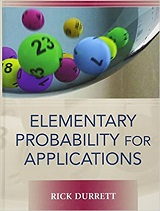Book Description

This clear and lively introduction to probability theory concentrates on the results that are the most useful for applications, including combinatorial probability and Markov chains.

Concise and focused, it is designed for a one-semester introductory course in probability for students who have some familiarity with basic calculus. Reflecting the author's philosophy that the best way to learn probability is to see it in action, there are more than 350 problems and 200 examples.

The examples contain all the old standards such as the birthday problem and Monty Hall, but also include a number of applications not found in other books, from areas as broad ranging as genetics, sports, finance, and inventory management.

The book has a nice interplay between probability modeling and scientific applications, whether from biology, sports, or discussions of China's one-child policy. Many of the examples are thought provoking, including ones on DNA samples for paternity cases and others about the O.J. Simpson trial. And the large selection of interesting problems builds basic skills and deepens or extends the main ideas.

• Rick Durrett received his Ph.D. in Operations Research from Stanford University in 1976. After nine years at UCLA and twenty-five at Cornell University, he moved to Duke University in 2010, where he is a Professor of Mathematics. He is the author of 8 books and more than 170 journal articles on a wide variety of topics, and he has supervised more than 40 Ph.D. students. He is a member of the National Academy of Science and the American Academy of Arts and Sciences and a Fellow of the Institute of Mathematical Statistics.
Reviews, Ratings, and Recommendations: Related Book Categories: Read and Download Links:Similar Books:
•Probability Theory: The Logic of Science (E. T. Jaynes)

Going beyond the conventional mathematics of probability theory, this study views the subject in a wider context. It discusses new results, along with applications of probability theory to a variety of problems.

•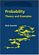Probability: Theory and Examples (Rick Durrett)

This lively introduction to measure-theoretic probability theory covers laws of large numbers, central limit theorems, random walks, martingales, Markov chains, ergodic theorems, and Brownian motion.

•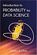Introduction to Probability for Data Science (Stanley Chan)

This book is an introductory textbook in undergraduate probability in the context of data science to emphasize the inseparability between data (computing) and probability (theory) in our time, with examples in both MATLAB and Python.

•Introduction to Probability, Statistics, and Random Processes

This book introduces students to probability, statistics, and stochastic processes. It provides a clear and intuitive approach to these topics while maintaining mathematical accuracy. The book contains a large number of solved exercises.

•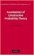Foundations of Constructive Probability Theory (Yuen-Kwok Chan)

This book provides a systematic and general theory of probability within the framework of Constructive Mathematics. It can serve as a parallel introduction into constructive mathematics and rigorous probability theory.

•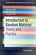Introduction to Random Matrices: Theory and Practice

This is a book for absolute beginners. The aim is to provide a truly accessible introductory account of Random Matrix theory. Most chapters are accompanied by MATLAB codes to guide readers through the numerical check of most analytical results.

•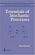Essentials of Stochastic Processes (Rick Durrett)

Stochastic processes have become important for many fields, including mathematical finance and engineering. Written by one of the worlds leading probabilists, this book presents recent results previously available only in specialized monographs.

•Introduction to Probability (Charles M. Grinstead, et al)

The book is a beautiful introduction to probability theory at the beginning level. The book contains a lot of examples and an easy development of theory without any sacrifice of rigor, keeping the abstraction to a minimal level.

•Probability Theory: The Logic of Science (E. T. Jaynes)

Going beyond the conventional mathematics of probability theory, this study views the subject in a wider context. It discusses new results, along with applications of probability theory to a variety of problems.

•Probability: Theory and Examples (Rick Durrett)

This lively introduction to measure-theoretic probability theory covers laws of large numbers, central limit theorems, random walks, martingales, Markov chains, ergodic theorems, and Brownian motion.

•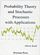Probability Theory and Stochastic Processes with Applications

This book provides an introduction to probability theory and discrete and continuous stochastic processes and its applications. It has a unique approach that provides a broad and wide introduction into the fascinating area of probability theory.

Book Categories
 :All CategoriesTop Free BooksRecent BooksMiscellaneous BooksComputer EngineeringComputer LanguagesComputer ScienceData Science/DatabasesJava and Java EE (J2EE)Linux and UnixMathematicsMicrosoft and .NETMobile ComputingNetworking and CommunicationsSoftware EngineeringSpecial TopicsWeb Programming
Other Categories